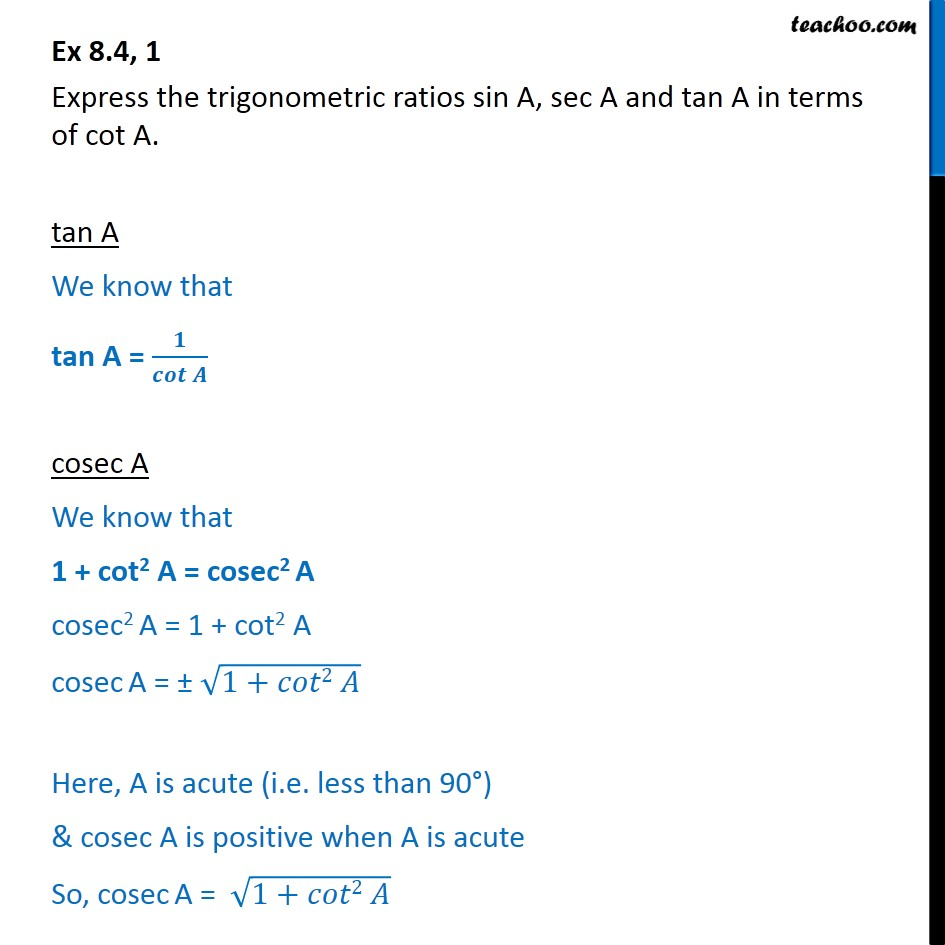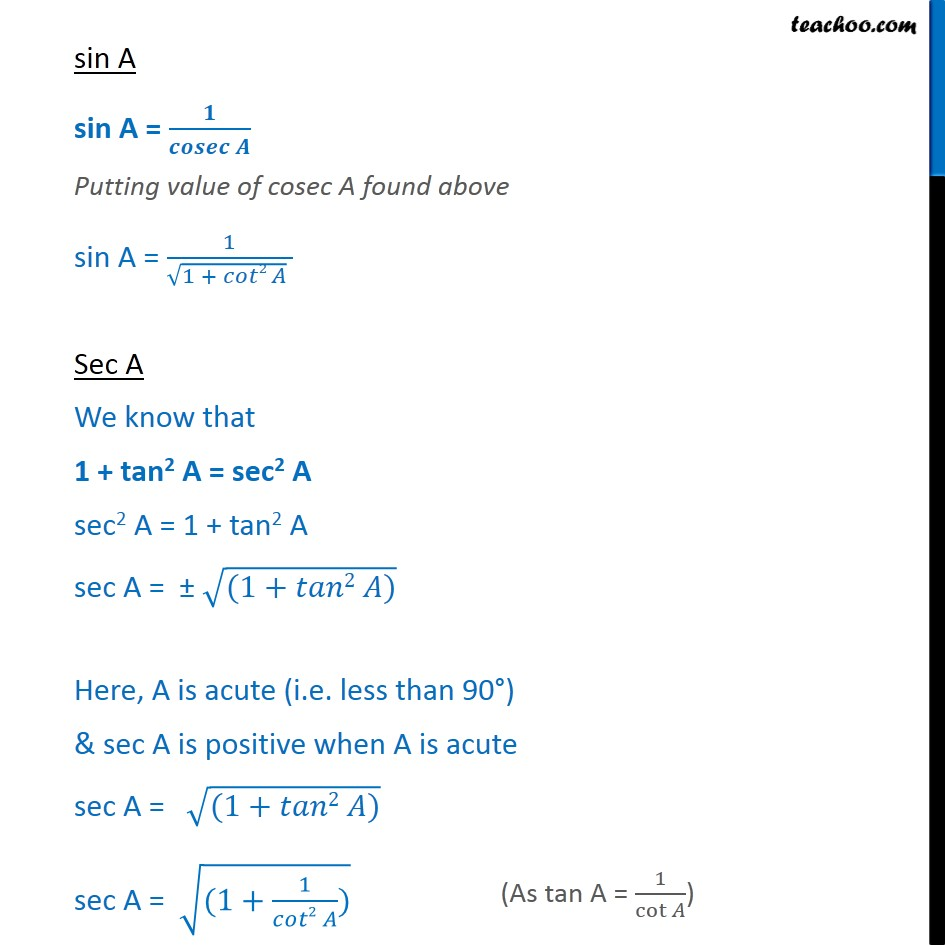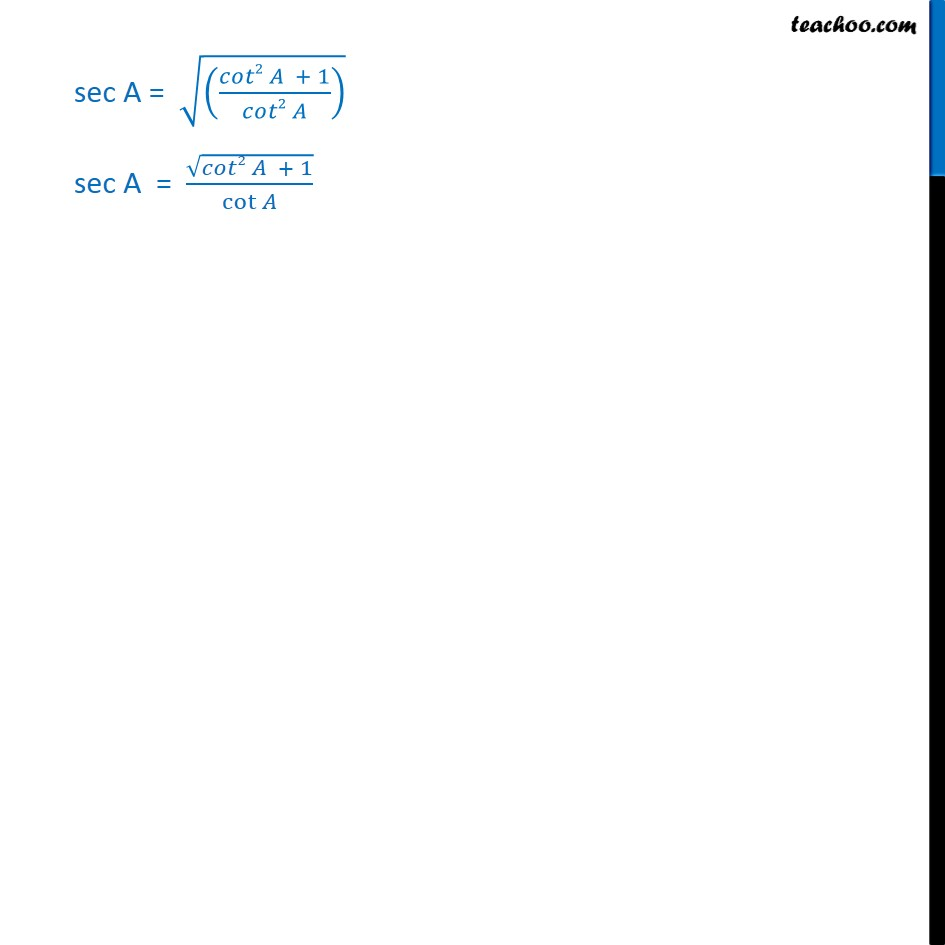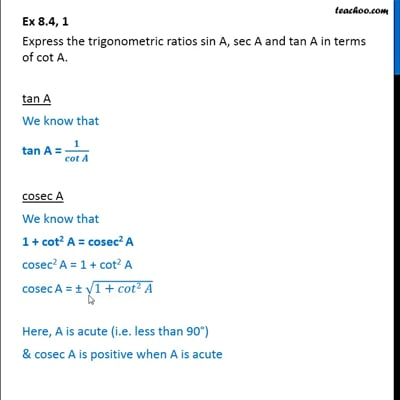Ex 8.4

Chapter 8 Class 10 Introduction to Trignometry
Serial order wiseThis video is only available for Teachoo black users

Maths Crash Course - Live lectures + all videos + Real time Doubt solving!

### Transcript

Ex 8.4, 1 Express the trigonometric ratios sin A, sec A and tan A in terms of cot A. tan A We know that tan A = 𝟏/𝒄𝒐𝒕⁡𝑨 cosec A We know that 1 + cot2 A = cosec2 A cosec2 A = 1 + cot2 A cosec A = ± √(1+𝑐𝑜𝑡2 𝐴) Here, A is acute (i.e. less than 90°) & cosec A is positive when A is acute So, cosec A = √(1+𝑐𝑜𝑡2 𝐴) sin A sin A = 𝟏/(𝒄𝒐𝒔𝒆𝒄 𝑨) Putting value of cosec A found above sin A = 1/(√(1 + 𝑐𝑜𝑡2 𝐴) ) Sec A We know that 1 + tan2 A = sec2 A sec2 A = 1 + tan2 A sec A = ± √((1+𝑡𝑎𝑛2 𝐴)) Here, A is acute (i.e. less than 90°) & sec A is positive when A is acute sec A = √((1+𝑡𝑎𝑛2 𝐴)) sec A = √((1+1/(𝑐𝑜𝑡2 𝐴))) sec A = √(((𝑐𝑜𝑡2 𝐴 + 1)/(𝑐𝑜𝑡2 𝐴)) ) sec A = √(𝑐𝑜𝑡2 𝐴 + 1)/cot⁡𝐴